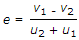# Civil Engineering - Applied Mechanics

### Exercise :: Applied Mechanics - Section 1

41.

A load of 500 kg was lifted through a distance of 13 cm. by an effort of 25 kg which moved through a distance of 650 cm. The efficiency of the lifting machine is

 A. 50% B. 40% C. 55% D. 30%.

Explanation:

No answer description available for this question. Let us discuss.

42.

Maximum efficiency of a screw jack for the angle of friction φ, is

 A.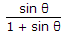B.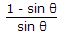C.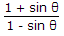D.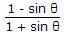Explanation:

No answer description available for this question. Let us discuss.

43.

A string of length 90 cm is fastened to two points A and B at the same level 60 cm apart. A ring weighing 120 g is slided on the string. A horizontal force P is applied to the ring such that it is in equilibrium vertically below B. The value of P is :

 A. 40 g B. 60 g C. 80 g D. 100 g.

Explanation:

No answer description available for this question. Let us discuss.

44.

The reaction at the support A of the beam shown in below figure is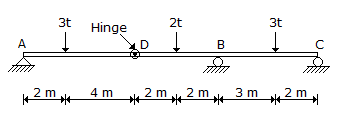A. 2 t B. 5.8 t C. 0.2 t D. 3.5 t.

Explanation:

No answer description available for this question. Let us discuss.

45.

u1 and u2 are the velocities of approach of two moving bodies in the same direction and their corresponding velocities of separation are v1 and v2. As per Newton's law of collision of elastic bodies, the coefficient of restitution (e) is given by

 A.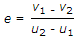B.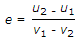C.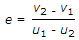D.